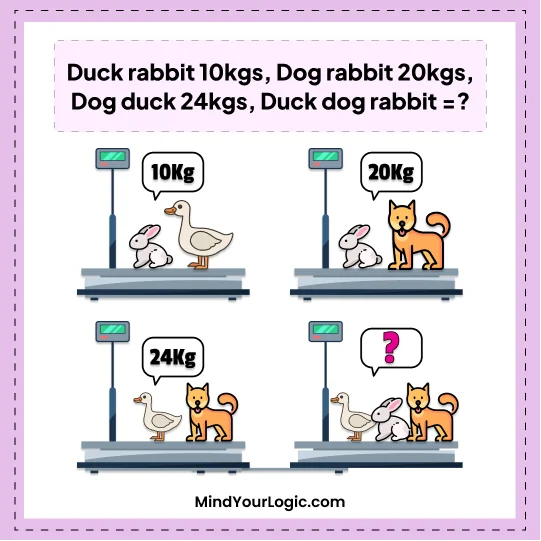# Duck Rabit Dog Weight-Math riddle

###### 66.Math Riddles
`Given that the weight of a Rabbit and a Duck is 10 kilograms, the weight of a Dog and a Rabbit is 20 kilograms, and the weight of a Dog and a Duck is 24 kilograms, what is the total weight of a Dog, a Rabbit, and a Duck?`• A.25
• B.35
• C.27
• D.40
• ```Explanation :

Let the duck be ‘Du’

The dog be ‘Do’

The rabbit be ‘R’.

From the above picture,

we can form equations as,

R + Du = 10kg.…equation (1)

R + Do = 20kg….equation (2)

Do + Du = 24kg…equation (3)

& R + Do + Du = ?

Rearranging the equations,

equation (1) = R + Du = 10kg

Du = 10kg – R

equation (2) = R + Do = 20kg

Do = 20kg – R

Putting the values of Do & Du in equation (3),

Do + Du = 24kg

20kg – R + 10kg – R = 24kg

30kg – 2R = 24kg

2R = 6

Rabbit, R = 3kg

Substituting the value of R, in equation (1) & equation (2)

We get,

equation (1), R + Du = 10kg

3 + Du = 10kg

Duck, Du = 7kg

Also, equation(2), R + Do = 20kg

3 + Do = 20kg

Dog, Do = 17kg.